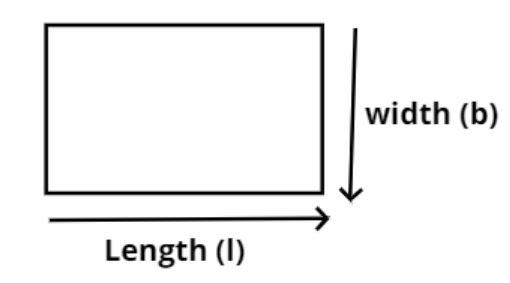Courses
Courses for Kids
Free study material
Free LIVE classes
MoreLIVE
Join Vedantu’s FREE Mastercalss

# The perimeter of a rectangle is 13 cm and its width is $2\dfrac{3}{4}{\text{ cm}}$. Find its length.Verified
359.4k+ views
Hint: In this question the perimeter of the rectangle is given to us and one of the sides is also known. So directly use the basic formula for the perimeter of the rectangle which is $s = 2\left( {l + b} \right)$where s is the perimeter, to obtain the unknown side.Given data
Perimeter (s) of the rectangle $= 13{\text{ cm}}$.
Width (b) of the rectangle $2\dfrac{3}{4}{\text{ cm}}$.
Now we have to calculate the length (l) of the rectangle.
So as we know that the perimeter of any shape is the sum of its all sides.
So from figure the perimeter of the rectangle is
$s = 2\left( {l + b} \right)$…………………… (1)
Now covert the width of the rectangle into improper fraction,
$b = 2\dfrac{3}{4} = \dfrac{{\left( {2 \times 4} \right) + 3}}{4} = \dfrac{{11}}{4}{\text{ cm}}$
Now substitute these values in equation (1) we have,
$s = 2\left( {l + b} \right)$
$\Rightarrow 13 = 2\left( {l + \dfrac{{11}}{4}} \right)$
Divide by 2 in above equation we have,
$\Rightarrow \dfrac{{13}}{2} = l + \dfrac{{11}}{4}$
Now simplify the above equation we have,
$\Rightarrow l = \dfrac{{13}}{2} - \dfrac{{11}}{4} = \dfrac{{26}}{4} - \dfrac{{11}}{4} = \dfrac{{15}}{4}{\text{ cm}}$
So, the length of the rectangle is$\dfrac{{15}}{4}{\text{ cm}}$.
So, this is the required answer.

Note: Whenever we face such types of problems the key concept is simply to have the good gist of the basic perimeter formula of rectangle. This understanding of direct formula will help you get on the right track to reach the required entity.
Last updated date: 16th Sep 2023
Total views: 359.4k
Views today: 5.59k